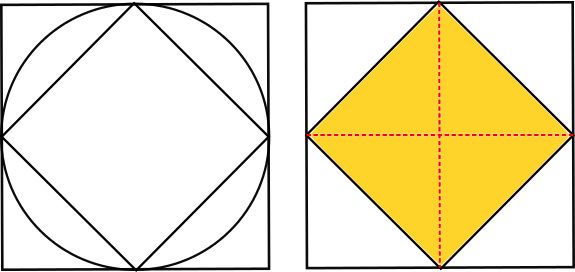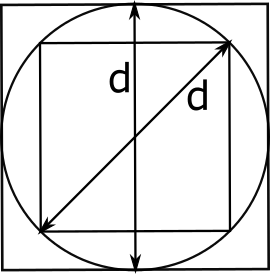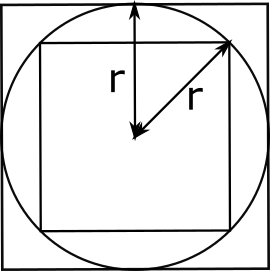#### You may also like### Dividing the Field

A farmer has a field which is the shape of a trapezium as illustrated below. To increase his profits he wishes to grow two different crops. To do this he would like to divide the field into two trapeziums each of equal area. How could he do this?### Two Circles

Draw two circles, each of radius 1 unit, so that each circle goes through the centre of the other one. What is the area of the overlap?### Star Gazing

Find the ratio of the outer shaded area to the inner area for a six pointed star and an eight pointed star.

# Square in a Circle in a Square

##### Age 14 to 16 Short Challenge Level:
Cutting up the squares to compare their areas
Rotating the smaller square so that its corners touch the sides of the larger square, and then removing the circle, gives the images shown below.It is clear from the image with the red dotted lines on it that the smaller square occupies half of the area of the larger square.

So the ratio of the area of the smaller square to the area of the larger square is 1:2.

Using relationships between the side lengths of the squares and the diameter of the circle
In the diagrams below, two diameters labelled d are drawn onto the circle, and then the squares are each shown individually with one of the diameters.The area of the larger square is d$^2$, and the area of the smaller square is s$^2$, so we want the ratio s$^2$:d$^2$.

We can use Pythagoras' theorem to find a relationship between s and d, because the smaller square can be split into two right-angled triangles.
d$^2$ = s$^2$ + s$^2$, so d$^2$ = 2s$^2$.
Which means that the ratio s$^2$:d$^2$ is 1:2.

Using relationships between the side lengths of the squares and the radius of the circle
In the diagram below, two radii of the circle are shown, both labelled $\text{r}$.The larger square has side length $2\text{r}$, so its area is $(2\text{r})^2=4\text{r}^2$.

The diagram below is helpful for finding $x$, which is half of the side length of the smaller square.By Pythagoras' theorem, $\text{r}^2=x^2 + x^2$, so $\text{r}^2 = 2x^2$, so $x^2$ = $\frac{1}{2}\text{r}^2$.

That means $x$=$\frac{1}{\sqrt{2}}\text{r}$, so the side length of the smaller square is $\frac{2}{\sqrt{2}}\text{r}$ (which is equal to $\sqrt{2}\text{r}$).

So the area of the smaller square is $\left(\frac{2}{\sqrt2}\text{r}\right)^2=\frac{4}{2}\text{r}^2=2\text{r}^2$.

So the ratio of the area of the smaller square to the area of the larger square is $2\text{r}^2$:$4\text{r}^2$, which simplifies to 1:2.
You can find more short problems, arranged by curriculum topic, in our short problems collection.